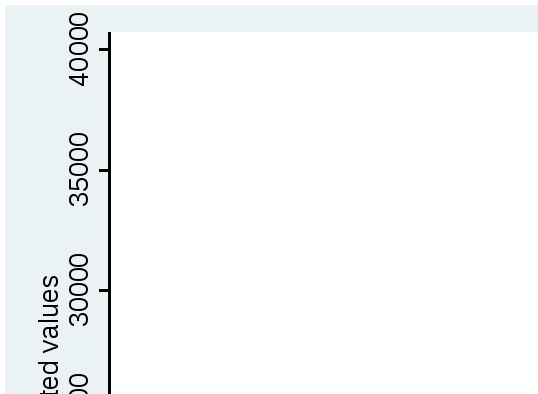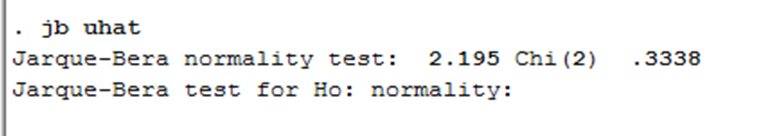# From the linear model we find expected u

 Mean: 28679.63452 ± 2*520.5117 => (27638.61112;29720.65792) Individual: 28679.63452 ± 2*2382.337 => (23914.96052;33444.30852) The assumption of normality of the disturbance is tested by several tests: qnorm, Qnorm test shows how normally our ui is distributed by the discretionary illustration in the graph, which is normal:The Jarque-Bera Test shows whether sample data is matching skewness and kurtosis with normal distribution:The probability is 0.3338, which is higher than 0.5, meaning than we accept H0, and say that there is normality. 00006550 Download 0.53 Mb.Do'stlaringiz bilan baham: